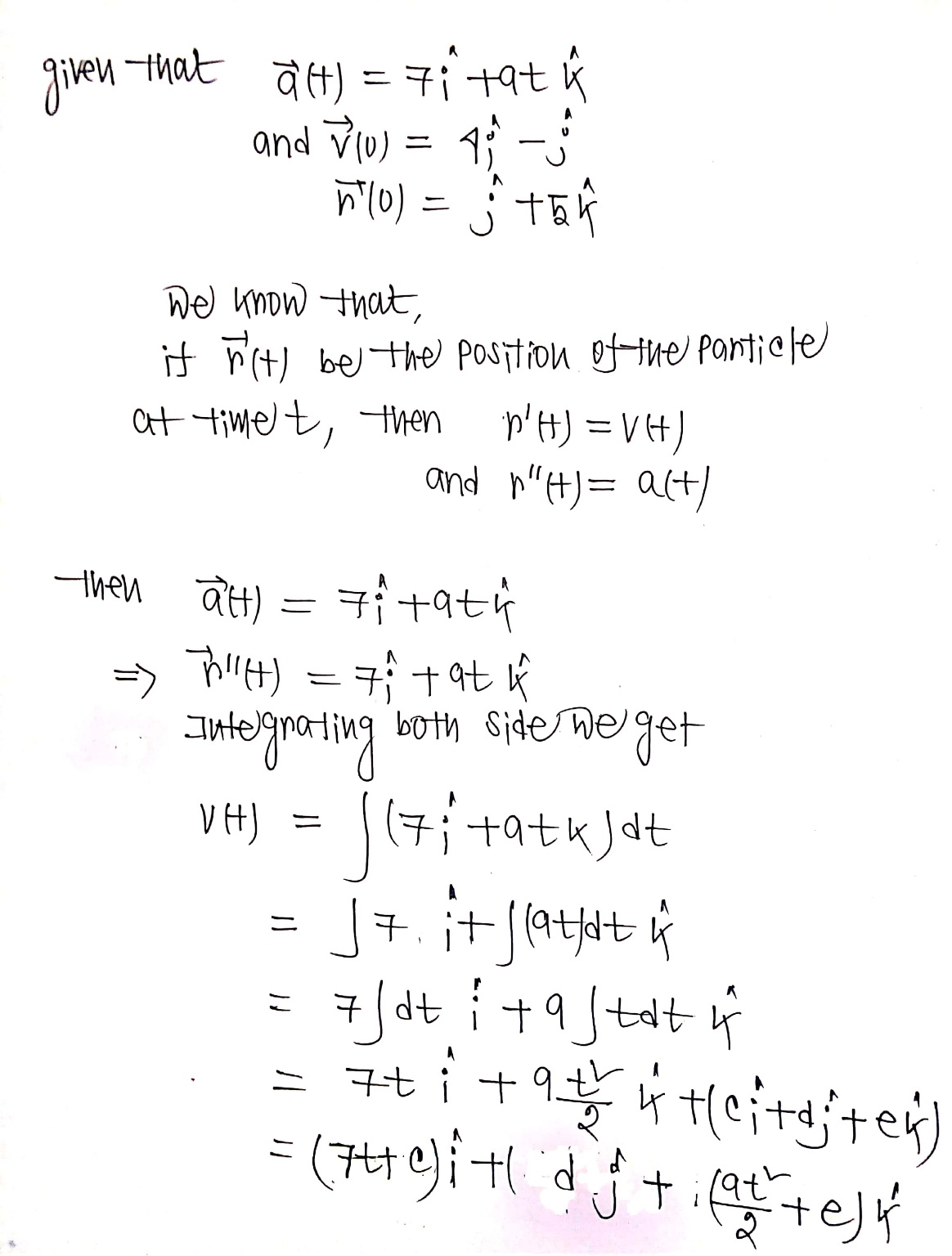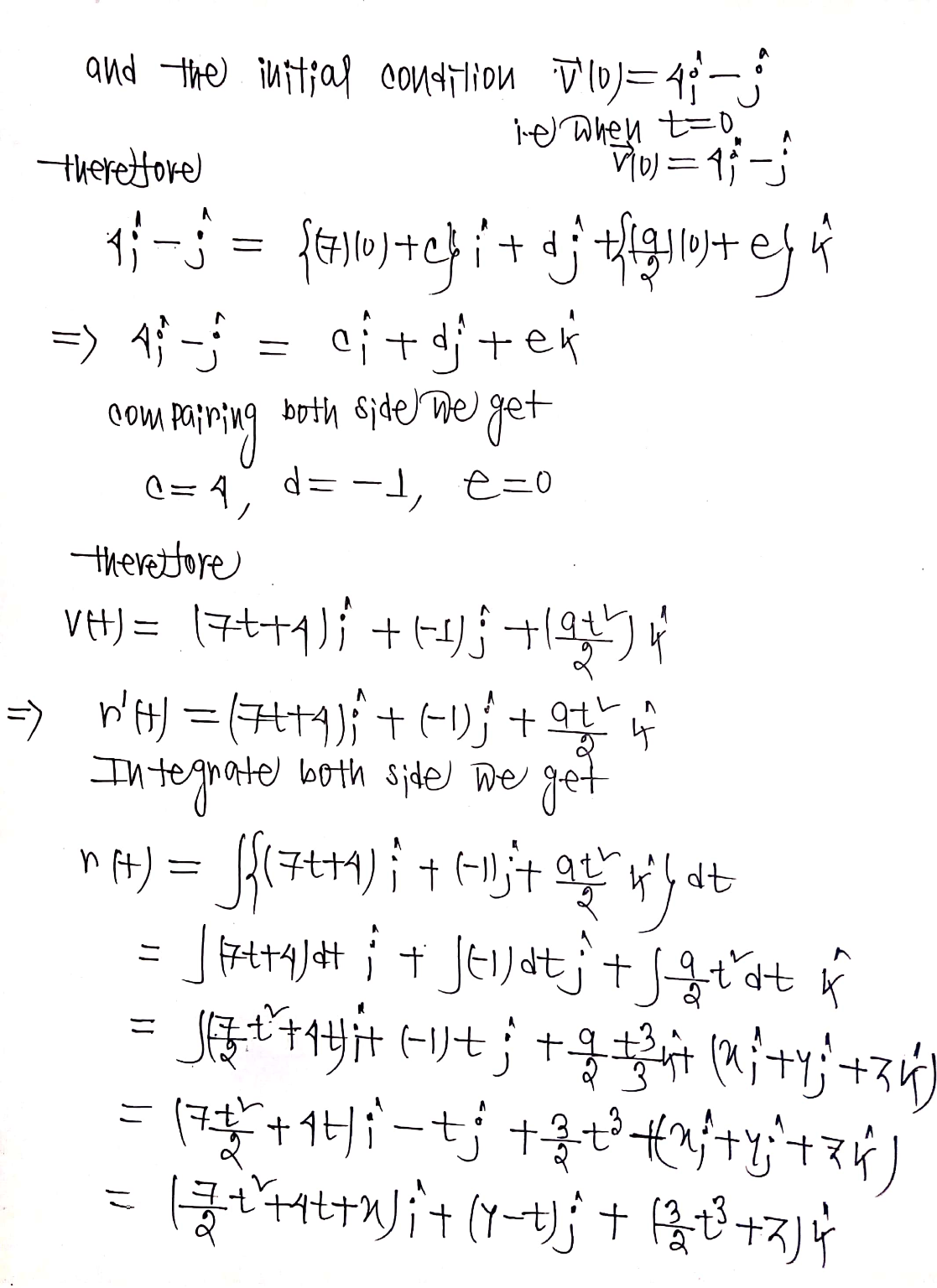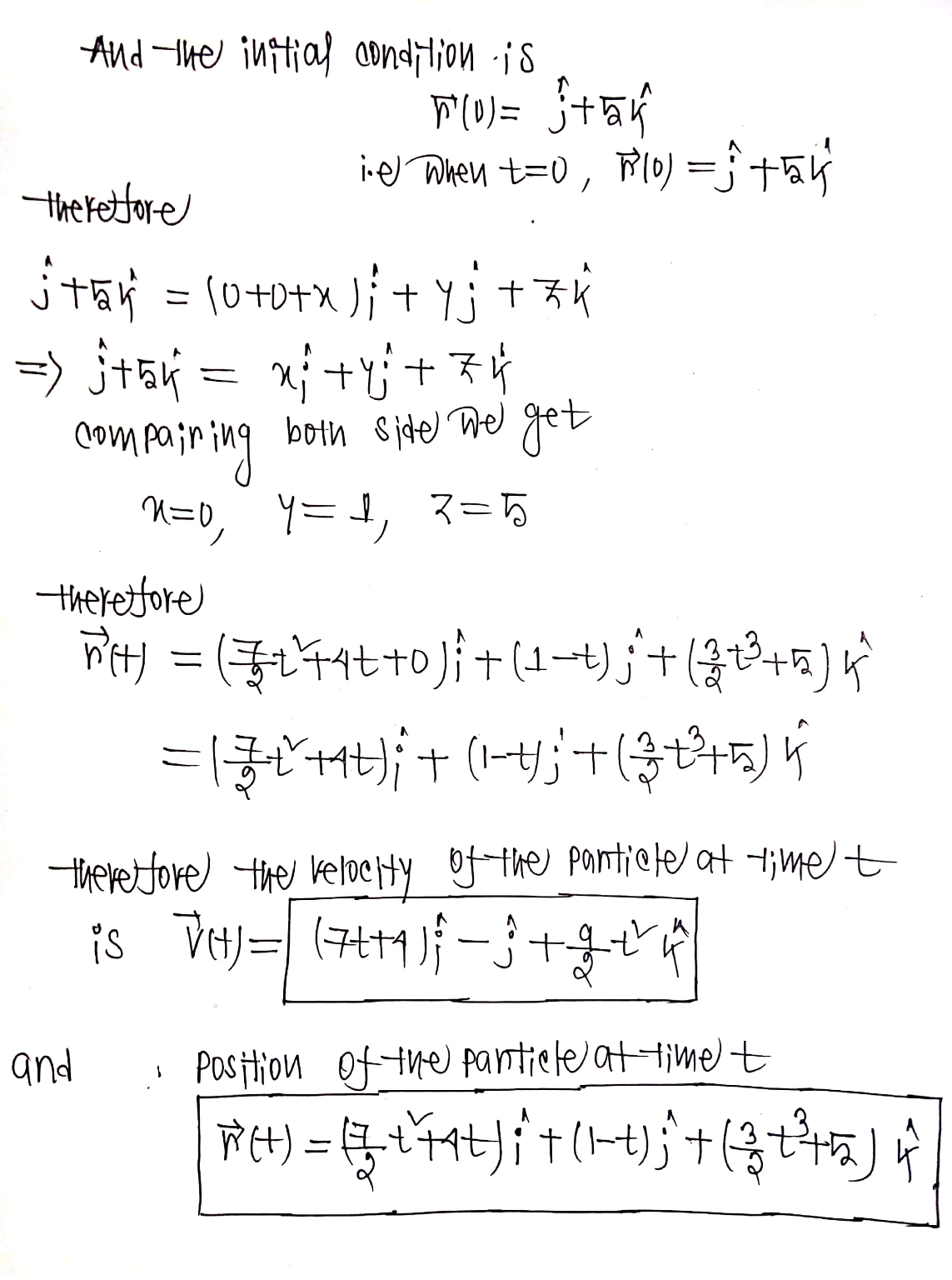In: Math

# Suppose that a particle has the following acceleration vector and initial velocity and position vectors. a(t)  ...

 Suppose that a particle has the following acceleration vector and initial velocity and position vectors. a(t)  =  7 i  +  9t k,    v(0)  =  4 i  −  j,    r(0)  =  j  +  5 k
 (a) Find the velocity of the particle at time t.
 (b) Find the position of the particle at time t.

## Solutions

##### Expert Solution## Related Solutions

##### 1) Find the velocity and position vectors of a particle that has the given acceleration and...
1) Find the velocity and position vectors of a particle that has the given acceleration and the given initial velocity and position. a(t) = 7i+ 4j,    v(0) = k,    r(0) = i 2) Find the tangential and normal components of the acceleration vector. r(t) = 5(3t − t3) i + 15t2 j
##### Find the velocity, acceleration, and speed of a particle with the given position function. r(t) =...
Find the velocity, acceleration, and speed of a particle with the given position function. r(t) = 2 cos(t), 2t, 2 sin(t)
##### Find the velocity, acceleration, and speed of a particle with the given position function. r(t) =...
Find the velocity, acceleration, and speed of a particle with the given position function. r(t) = 9 cos(t), 8 sin(t) v(t) = a(t) = |v(t)| = Sketch the path of the particle and draw the velocity and acceleration vectors for t = π 3 .
##### write a matlab code to find the following: initial position, initial velocity, and acceleration using the...
write a matlab code to find the following: initial position, initial velocity, and acceleration using the algorithm and information below time(seconds). height(m) velocity(m/s) 0. 0.2. 2.95 algorithm: 1. Enter data in to arrays. 2. Fit the height data to a 2nd order polynomial. 3. Evaluate the polynomial at enough points to get a smooth curve. 4. Find the velocity model by taking derivative of the height polynomial. 5. Evaluate the velocity polynomial at enough times to get a smooth curve
##### A particle moves with acceleration function a(t) = 2x+3. Its initial velocity is v(0) = 2...
A particle moves with acceleration function a(t) = 2x+3. Its initial velocity is v(0) = 2 m/s and its initial displacement is s(0) = 5 m. Find its position after t seconds.
##### The position vector F(t) of a moving particle at time t[s] is given by F(t)= e^t...
The position vector F(t) of a moving particle at time t[s] is given by F(t)= e^t sin(t)i-j+e^t cos(t)k a) Calculate the acceleration a(t). b) Find the distance traveled by the particle at time t = 3π/2, if the particle starts its motion at time t = π/2. c) Find the unit tangent vector of this particle at time t = 3π/2. d) Find the curvature of the path of this particle at time t = 3π/2.
##### (1 point) For the given position vectors r(t)r(t) compute the unit tangent vector T(t)T(t) for the...
(1 point) For the given position vectors r(t)r(t) compute the unit tangent vector T(t)T(t) for the given value of tt . A) Let r(t)=〈cos5t,sin5t〉 Then T(π4)〈 B) Let r(t)=〈t^2,t^3〉 Then T(4)=〈 C) Let r(t)=e^(5t)i+e^(−4t)j+tk Then T(−5)=
##### Answer the following questions for an orbit with the following Position and Velocity vectors: (R =...
Answer the following questions for an orbit with the following Position and Velocity vectors: (R = 7626I + 4972J + 806K km, V = 0.626I - 0.690J - 6.160K km/s) 1. Determine the size of the size (semimajor axis) of this orbit. 2. Determine the shape (eccentricity) of this orbit. 3. Determine the inclination of this orbit. 4. Determine the right ascension of the ascending node for this orbit. 5. Determine the argument of the perigee for this orbit. 6....
##### 1. Describe the Velocity vector and Acceleration vector of ball being thrown straight up in the...
1. Describe the Velocity vector and Acceleration vector of ball being thrown straight up in the air and then coming back down. 2. A tennis ball is being swung around in a circle in uniform circular motion. Describe the direction of the linear velocity vector, the linear acceleration vector, and the centripetal (radial) acceleration vector. 3. Which pulls harder gravitationally, the Earth on the Moon, or the Moon on the Earth? 4. If I double the force I apply pushing...
##### the position of a particle when t=0 is 9.0m and its velocity is 3.0 m/s. from...
the position of a particle when t=0 is 9.0m and its velocity is 3.0 m/s. from t=0 to t=3.5s the acceleration of a particle is a= 8 + 6t + 3t^2 + t^3 m/s^2 . From t=3.5s until it comes to rest, its acceleration is a= -8 m/s^2 . Determine the total travel time and the total distance.